# Solving Quadratic Equations 9 6 by Factoring Warm

• Slides: 14Solving Quadratic Equations 9 -6 by Factoring Warm Up Find each product. 1. (x + 2)(x + 7) 2. (x – 11)(x + 5) x 2 + 9 x + 14 x 2 – 6 x – 55 3. (x – 10)2 x 2 – 20 x + 100 Factor each polynomial. (x + 5)(x + 7) 4. x 2 + 12 x + 35 (x – 7)(x + 9) 5. x 2 + 2 x – 63 6. x 2 – 10 x + 16 (x – 2)(x – 8) 7. 2 x 2 – 16 x + 32 2(x – 4)2 Holt Algebra 1Solving Quadratic Equations 9 -6 by Factoring Learning Target Students will be able to: Solve quadratic equations by factoring. Holt Algebra 1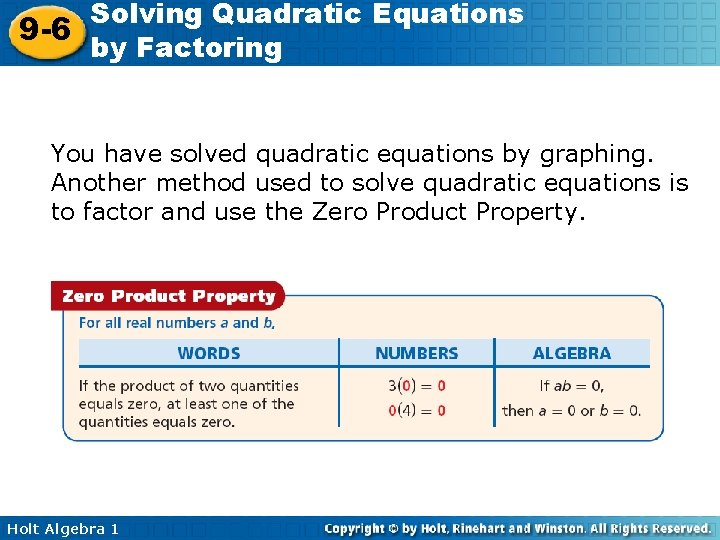Solving Quadratic Equations 9 -6 by Factoring You have solved quadratic equations by graphing. Another method used to solve quadratic equations is to factor and use the Zero Product Property. Holt Algebra 1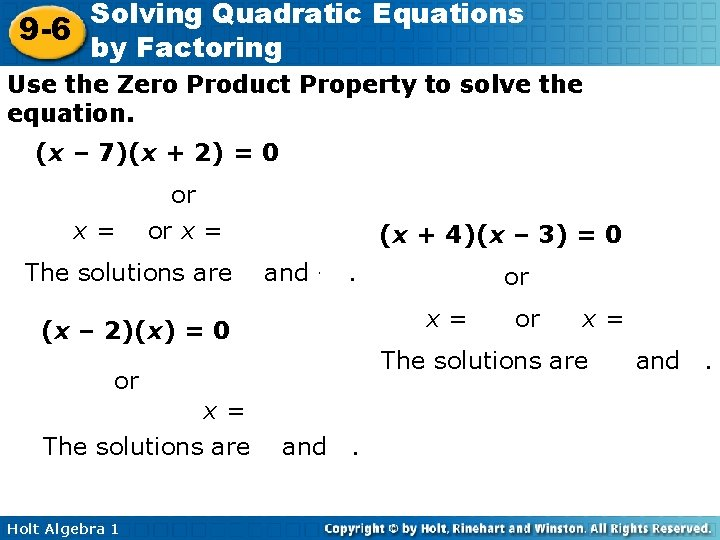Solving Quadratic Equations 9 -6 by Factoring Use the Zero Product Property to solve the equation. (x – 7)(x + 2) = 0 x – 7 = 0 or x + 2 = 0 x = 7 or x = – 2 (x + 4)(x – 3) = 0 The solutions are 7 and – 2. x + 4 = 0 or x – 3 = 0 (x – 2)(x) = 0 x = 0 or x – 2 = 0 x=2 The solutions are 0 and 2. Holt Algebra 1 x = – 4 or x=3 The solutions are – 4 and 3.Solving Quadratic Equations 9 -6 by Factoring If a quadratic equation is written in standard form, ax 2 + bx + c = 0, then to solve the equation, you may need to factor before using the Zero Product Property. Helpful Hint To review factoring techniques, see lessons 8 -3 through 8 -5. Holt Algebra 1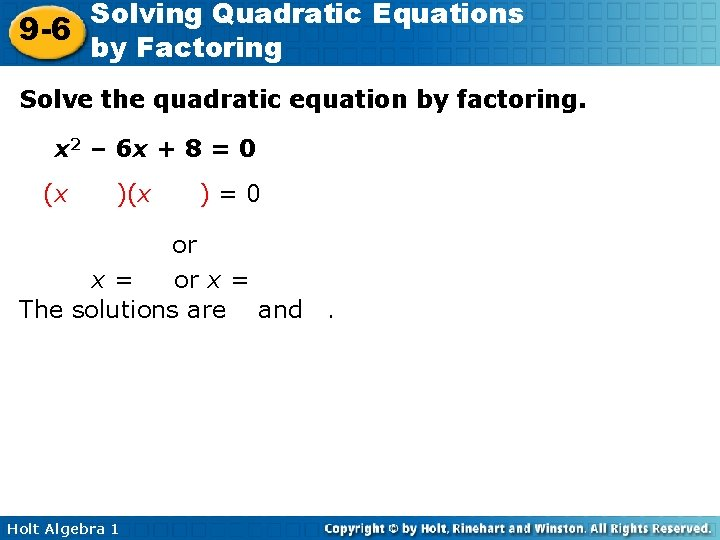Solving Quadratic Equations 9 -6 by Factoring Solve the quadratic equation by factoring. x 2 – 6 x + 8 = 0 (x – 4)(x – 2) = 0 x – 4 = 0 or x – 2 = 0 x = 4 or x = 2 The solutions are 4 and 2. Holt Algebra 1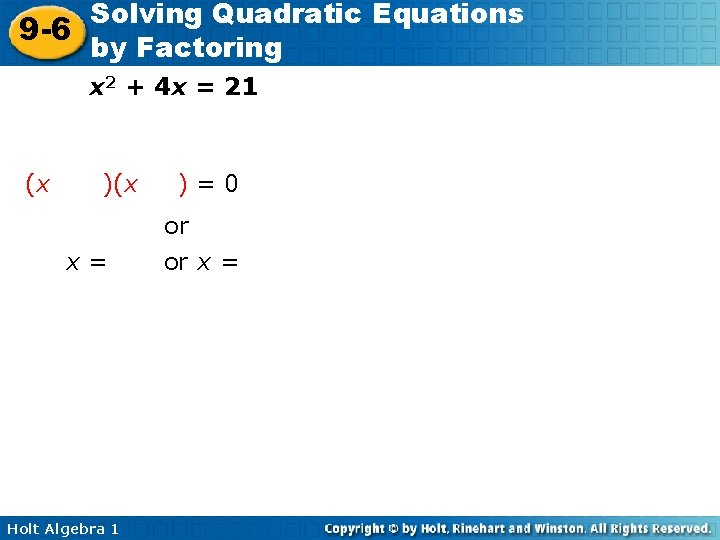Solving Quadratic Equations 9 -6 by Factoring xx 22 + 4 x = = 21 21 – 21 x 2 + 4 x – 21 = 0 (x + 7)(x – 3) = 0 x + 7 = 0 or x – 3 = 0 x = – 7 or x = 3 The solutions are – 7 and 3. Holt Algebra 1Solving Quadratic Equations 9 -6 by Factoring Solve the quadratic equation by factoring. 2 = 20 x + 50 – 2 x 2 = 20 x + 50 +2 x 2 0 = 2 x 2 + 20 x + 50 0 = 2(x 2 + 10 x + 25) 0 = 2(x + 5) x+5=0 Holt Algebra 1 x = – 5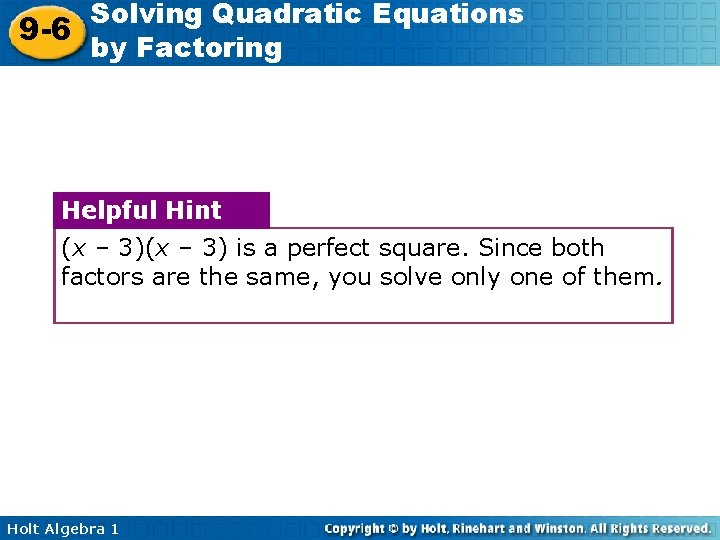Solving Quadratic Equations 9 -6 by Factoring Helpful Hint (x – 3) is a perfect square. Since both factors are the same, you solve only one of them. Holt Algebra 1Solving Quadratic Equations 9 -6 by Factoring Solve the quadratic equation by factoring. Check your answer. x 2 – 6 x + 9 = 0 (x – 3) = 0 x– 3=0 x=3 Holt Algebra 1Solving Quadratic Equations 9 -6 by Factoring Solve the quadratic equation by factoring. x 22 + 4 x 4 x = = 55 – 5 x 2 + 4 x – 5 = 0 (x – 1)(x + 5) = 0 x – 1 = 0 or x + 5 = 0 x=1 or x = – 5 The solutions are 1 and – 5. Holt Algebra 1Solving Quadratic Equations 9 -6 by Factoring Solve the quadratic equation by factoring. 30 x = – 9 x 2 – 25 9 x 2 + 30 x + 25 = 0 (3 x + 5) = 0 3 x + 5 = 0 Holt Algebra 1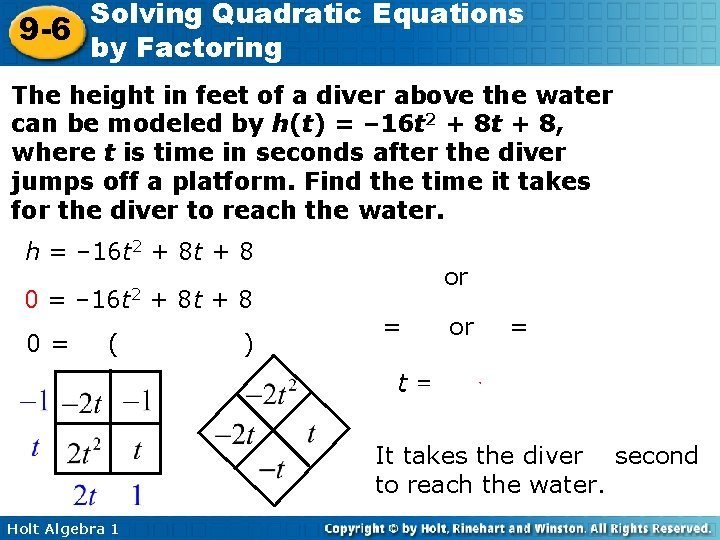Solving Quadratic Equations 9 -6 by Factoring The height in feet of a diver above the water can be modeled by h(t) = – 16 t 2 + 8 t + 8, where t is time in seconds after the diver jumps off a platform. Find the time it takes for the diver to reach the water. h = – 16 t 2 + 8 t + 8 0= – 8(2 t 2 – t – 1) 2 t + 1 = 0 or t – 1= 0 2 t = – 1 or t = 1 It takes the diver 1 second to reach the water. Holt Algebra 1Solving Quadratic Equations 9 -6 by Factoring HW pp. 633 -635/20 -44, 46 -50, 56 -67 Holt Algebra 1